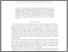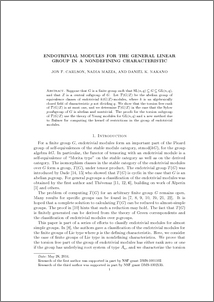# Endotrivial modules for the general linear group in a nondefining characteristic

Carlson, Jon and Mazza, Nadia and Nakano, Daniel (2014) Endotrivial modules for the general linear group in a nondefining characteristic. Mathematische Zeitschrift, 278 (3-4). pp. 901-925. ISSN 0025-5874Preview
PDF (gl-rev6)
gl_rev6.pdf - Accepted Version

## Abstract

Suppose that \$G\$ is a finite group such that \$\SL(n,q)\subseteq G \subseteq \GL(n,q)\$, and that \$Z\$ is a central subgroup of \$G\$. Let \$T(G/Z)\$ be the abelian group of equivalence classes of endotrivial \$k(G/Z)\$-modules, where \$k\$ is an algebraically closed field of characteristic~\$p\$ not dividing \$q\$. We show that the torsion free rank of \$T(G/Z)\$ is at most one, and we determine \$T(G/Z)\$ in the case that the Sylow \$p\$-subgroup of \$G\$ is abelian and nontrivial. The proofs for the torsion subgroup of \$T(G/Z)\$ use the theory of Young modules for \$\GL(n,q)\$ and a new method due to Balmer for computing the kernel of restrictions in the group of endotrivial modules.

Item Type:
Journal Article
Journal or Publication Title:
Mathematische Zeitschrift
The original publication is available at www.link.springer.com
Uncontrolled Keywords:
/dk/atira/pure/subjectarea/asjc/2600
Subjects:
Departments:
ID Code:
69712
Deposited By:
Deposited On:
18 Jun 2014 07:48
Refereed?:
Yes
Published?:
Published